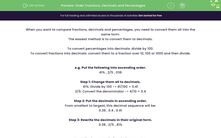# Order Fractions, Decimals and Percentages

In this worksheet, students will put groups of fractions, decimals and percentages into ascending or descending orders by converting them into decimals for easier comparison.Key stage:  KS 4

Year:  GCSE

GCSE Subjects:   Maths

GCSE Boards:   Pearson Edexcel, OCR, Eduqas, AQA,

Curriculum topic:   Number, Fractions, Decimals and Percentages

Curriculum subtopic:   Structure and Calculation Ordering Fractions, Decimals and Percentages

Popular topics:   Decimals worksheets

Difficulty level:#### Worksheet Overview

When you want to compare fractions, decimals and percentages, you need to convert them all into the same form.

The easiest method is to convert them to decimals.

To convert percentages into decimals: divide by 100.

To convert fractions into decimals: convert them to a fraction over 10, 100 or 1000 and then divide.

e.g. Put the following into ascending order.

41% , 2/5 , 038

Step 1: Change them all to decimals.

41%: Divide by 100 -> 41/100 = 0.41

2/5: Convert the denominator -> 4/10 = 0.4

Step 2: Put the decimals in ascending order.

From smallest to largest, this decimal sequence will be:

0.38 , 0.4 , 0.41

Step 3: Rewrite the decimals in their original form.

0.38 , 2/5 , 41%

Now let's give some similar challenges a try.

IMPORTANT: In this activity, write fractions in the form a/b and do not leave a space between a number and a percentage sign.

### What is EdPlace?

We're your National Curriculum aligned online education content provider helping each child succeed in English, maths and science from year 1 to GCSE. With an EdPlace account you’ll be able to track and measure progress, helping each child achieve their best. We build confidence and attainment by personalising each child’s learning at a level that suits them.

Get started